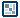## Question of the WeekPerspective in Flash
by ilyas usal

Introduction

First I'd like to thank Keith Peters from bit-101 for introducing me to perspective in Flash. Be sure to check his site, it's one of the best experimental sites out there.

We are going to see in this tutorial how you can give a realistic perspective effect to an object. I'm not going to lie to you: this is not very easy. But it is less hard than you could imagine. Even though it is not math-free, this tutorial will not get into very complex mathematic manipulations, but rather explain as simply as possible how it works. Then, when we feel comfortable about it, I will show you a few experiments I made with this, explaining step by step how I got there.

Let's take a look at what we know already about perspective. Basically, the farther the object, the smaller it looks. Good. The second thing is: ultimately, when you look far far away, all the objects seem to head towards one point on the horizon (imagine a very long tree lane). Let's call this point the horizon point (because I have no clue how it is called in English). Very well: we're ready to start.

A little bit of theory

Ever heard of Thales Theorem? No? Well it's very simple. And very useful. But first let's have a look at that picture. On the left, your eye, on the right, an object, and in the middle an imaginary plan that holds an image of the object, smaller than the actual object. This is the focal plan.[ this is a triangle (everybody follows?) ]

Let's introduce a very important quantity: the focal length (fl). This expression is borrowed from optics, and you could see it like this: imagine that you look out your window. Imagine also that nothing moves out there. You know that the closer from the window the objects will be, the bigger they will appear. That's the 'general' perspective. But there's more. Because the size of the objects depends also on where YOU stand relatively to the window. So basically, the focal length is the distance between you and that window. Therefore, a short focal length means you have your nose on the window. On the opposite, a long focal length means that you look at it from a long distance. This is about as clearly as I can explain it.

z is then how deep you go behind the window (z=0 is the window), the 'general' depth.

But I told you about Thales theorem. It is very useful for us to find the scaling formula: if we call `H` the real height of the object, and `h` the height that we see on the screen, then we have this relation:

`H/h=(fl+z)/fl`

Actually, we're looking for `h/H`, so the scaling factor is `fl/(fl+z)`.

Let's look at this for one second. Imagine that you have a long focal length (fl=1000). If you're on the screen, z=0 and the scaling factor equals to 1000/(1000+0)=1. The object's scale remains the same.
Now if z=500, the scaling factor equals to 1000/(1000+500)=0.66. The objects gets smaller.
Last thing to notice: the bigger the focal lenght, the less influence z has on the scale. For instance, let's find when the object's scale is 0.5. If fl=1000, then you must have z=2000. That's a lot. Now if fl=100, you need z=200. It comes much quicker.

Enough theory! Let's code!

First experiment

[ now this is a circle ]

We will create a ball that goes deeper and deeper.

1. Draw a circle with the circle tool. Make it quite large, so that it takes time to disappear. Then turn it into a movie clip (Ctrl+F8) and give it the instance name ball.

2. Open the Actions panel, and enter this code:
3. ```onClipEvent (load) {    z=0;    zspeed=5;    fl=300; } onClipEvent(enterFrame) {    scale=fl/(fl+z);    _xscale=_yscale=100*scale;    z+=zspeed; }```

4. The dot is getting smaller as its z position increases. Well, you're now halfway through! OK, maybe not halfway, but if you've understood that piece of code, everything else is a walk in the park. So let's explain it.
The variables are pretty straightforward:
1. z is the z position, 0 means on the screen.
2. zspeed is the speed of the dot.
3. fl is the focal length

You're encouraged to change these values to see what they actually mean (you can try a negative speed for instance).

Then we calculate the scale (it changes because z changes), and set the object to the right scale. Finally, we "move" the object by `zspeed` (in fact we don't really move it, we just increase its depth).

5. OK, this is very nice, but the dot doesn't go to the 'horizon point', its size simply decreases. In fact, we just considered that the ball and the horizon were aligned. This is quite simple to fix. All we have to do is choose a horizon point, and consider the offset of the ball (I called it `offx` here) as an object which size decreases just as any other object.

6. [ i marked the horizon point with a white spot ]

```onClipEvent (load) {    z=0;    zspeed=5;    fl=300;    xcenter=175;    ycenter=175;    offx=(_x-xcenter);    offy=(_y-ycenter); } onClipEvent(enterFrame) {    scale=fl/(fl+z);    _x=xcenter+offx*scale;    _y=ycenter+offy*scale;    _xscale=_yscale=100*scale;    z+=zspeed; }```

7. As you can see, all I did was rescale offx and offy, and then position my movie clip. To improve the movie, you can try to mess with the alpha of the clip a bit. For instance, at the end of the enterFrame:

`_alpha--;`

There! This is the end of our introduction to perspective. I hope it made sense. Be sure to check the sequel of this tutorial for more cool perspective effects (and more coding, yeah!!!).